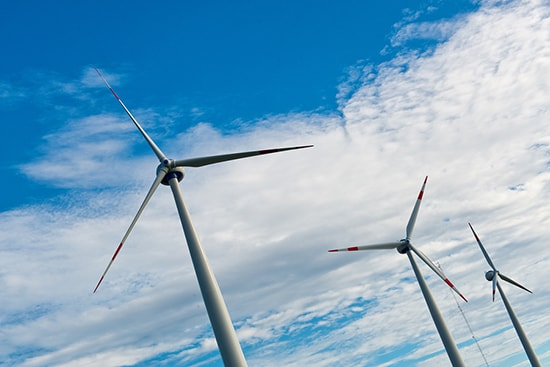Energy is a common word used by most people to mean strength and vitality. You hear people talking about it all the time, such as “I have no energy to do that”, “We need more renewable energy”, and “We must decrease our dependence on non-renewable energy”. But in physics, energy has a specific meaning. It is defined as the capacity to do work.
The Meaning of Work
In order to grasp the concept of energy, it is important to understand what work means. When you apply some force to an object to displace it through a certain distance, it is said that you have done some work. Examples of work in everyday life include moving a chair from one place to another or a worker lifting a load.
In order for work to be done, some force must be applied to an object, the object must be displaced through a certain distance, and there must be a cause. So work has three key ingredients – force, displacement and cause. This is mathematically expressed using the equation W = F * d (for a constant force in the direction of motion), where W is work, F is the force applied and d is the displacement.
Types of Energy
Now that you know that energy is the capacity to do work, it is time to learn about the two types of energy that exists in nature.

• Potential energy
The energy stored in an object due to its position. There are two forms of potential energy: gravitational potential energy and elastic potential energy.
• Kinetic energy
The energy possessed by a body in motion. It is represented by the following mathematical formula: KE = 0.5 x m x v^2, where m = mass of the object and v = speed of the object.

Forms of Energy
The second law of thermodynamics states that energy can neither be created nor destroyed, it can only be changed from one form to another. Thus, energy exists in many different forms in nature, which include:

• Thermal energy (heat)
The energy generated by the movement of the molecules of an object.
The only form of energy that we can see with our eyes.
• Chemical energy
The energy that is stored in the bonds of atoms and molecules.
• Electrical energy
The energy generated by electric fields.
• Magnetic energy
The energy generated by magnetic fields.
• Mechanical energy
This is the sum of potential energy and kinetic energy (TME = PE + KE).
• Soundwave
The energy propagated by a sound wave.
• Elastic energy
The energy that comes from the deformation of a material, exhibiting a restorative force
• Mechanical wave
The energy that is propagated by a deformational wave through an elastic material.
• Gravitational energy
The energy that comes from the gravitational field of large bodies, such as planets and stars.
• Ionization energy
The energy that is generated during the binding of an electron to an atom or a molecule.
• Nuclear energy
The energy that is generated during nuclear reactions or during the binding of nucleons to form the atomic nucleus.

These are just the basics of energy and there is so much more to this concept. If you find yourself struggling with this concept or simply want to improve yourself in this area, consider taking up a physics tuition. Your tutors will be able to break down this knowledge in a more comprehensive way and provide extra practices to anchor your concepts down.